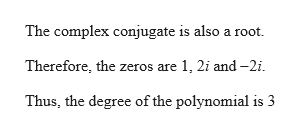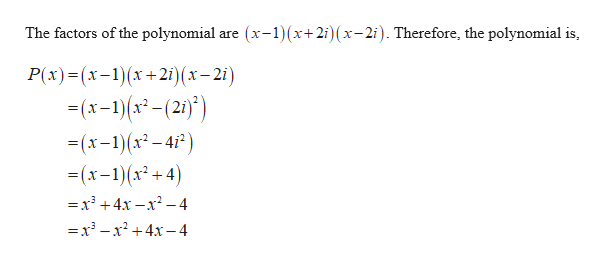# Find the function that has an output of 16 when x=2, and has zeros 1 and −2i.

Question
414 views

Find the function that has an output of 16 when x=2, and has zeros 1 and −2i.

check_circle

star
star
star
star
star
1 Rating
Step 1help_outlineImage TranscriptioncloseThe complex conjugate is also a root. Therefore, the zeros are 1, 2i and -2i. Thus, the degree of the polynomial is 3 fullscreen
Step 2help_outlineImage Transcriptionclose(x-1(x+2i) x-2i). Therefore, the polynomial is The factors of the polynomial are P(x)(x-1)(x+2i) (x-2i) -x-1)(x(2 =(x-1)(x-4) (x-1)x+4) =x3 +4x -x2-4 =x3 -x2+4x-4 fullscreen

### Want to see the full answer?

See Solution

#### Want to see this answer and more?

Solutions are written by subject experts who are available 24/7. Questions are typically answered within 1 hour.*

See Solution
*Response times may vary by subject and question.
Tagged in